NEET  >  NCERT Solutions: Kinetic Theory

# Kinetic Theory NCERT Solutions | Physics Class 11 - NEET

 1 Crore+ students have signed up on EduRev. Have you?

NCERT QUESTION

(Kinetic Theory)
Qns 13.1: Estimate the fraction of molecular volume to the actual volume occupied by oxygen gas at STP. Take the diameter of an oxygen molecule to be 3Å.
Ans: Diameter of an oxygen molecule, d = 3Å
Radius, r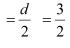= 1.5 Å = 1.5 × 10–8 cm
Actual volume occupied by 1 mole of oxygen gas at STP = 22400 cm3
Molecular volume of oxygen gas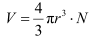Where, N is Avogadro’s number = 6.023 × 1023 molecules/mole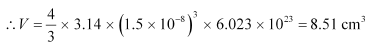Ratio of the molecular volume to the actual volume of oxygen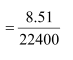= 3.8 × 10–4

Qns 13.2: Molar volume is the volume occupied by 1 mol of any (ideal) gas at standard temperature and pressure (STP: 1 atmospheric pressure, 0 °C). Show that it is 22.4 litres.
Ans: The ideal gas equation relating pressure (P), volume (V), and absolute temperature (T) is given as:

PV = nRT
Where,
R is the universal gas constant = 8.314 J mol–1 K–1
n = Number of moles = 1
T = Standard temperature = 273 K
P = Standard pressure = 1 atm = 1.013 × 105 Nm–2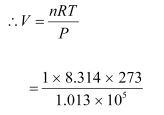= 0.0224 m3
= 22.4 litres
Hence, the molar volume of a gas at STP is 22.4 litres.

Qns 13.3: Figure 13.8 shows plot of PV/T versus P for 1.00×10–3 kg of oxygen gas at two different temperatures.
(a) What does the dotted plot signify?
(b) Which is true: T1 > T2 or T1 < T2?
(c) What is the value of PV/T where the curves meet on the y-axis?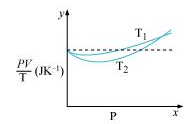(d) If we obtained similar plots for 1.00 ×10–3 kg of hydrogen, would we get the same value of PV/T at the point where the curves meet on the y-axis? If not, what mass of hydrogen yields the same value of PV/T (for low pressure high temperature region of the plot)? (Molecular mass of H2 = 2.02 u, of O2 = 32.0 u, R = 8.31 J mo1–1 K–1.)
Ans: (a) The dotted plot in the graph signifies the ideal behaviour of the gas, i.e., the ratio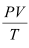is equal. μR (μ is the number of moles and R is the universal gas constant) is a constant quality. It is not dependent on the pressure of the gas.

(b) The dotted plot in the given graph represents an ideal gas. The curve of the gas at temperature T1 is closer to the dotted plot than the curve of the gas at temperature T2. A real gas approaches the behaviour of an ideal gas when its temperature increases. Therefore, T1 > T2 is true for the given plot.

(c) The value of the ratio PV/T, where the two curves meet, is μR. This is because the ideal gas equation is given as:

PV = μRT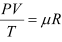Where,
P is the pressure
T is the temperature
V is the volume
μ is the number of moles
R is the universal constant
Molecular mass of oxygen = 32.0 g
Mass of oxygen = 1 × 10–3 kg = 1 g
R = 8.314 J mole–1 K–1
∴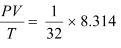= 0.26 J K–1
Therefore, the value of the ratio PV/T, where the curves meet on the y-axis, is
0.26 J K–1.

(d) If we obtain similar plots for 1.00 × 10–3 kg of hydrogen, then we will not get the same value of PV/T at the point where the curves meet the y-axis. This is because the molecular mass of hydrogen (2.02 u) is different from that of oxygen (32.0 u).
We have: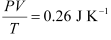R = 8.314 J mole–1 K–1
Molecular mass (M) of H2 = 2.02 u
m = Mass of H2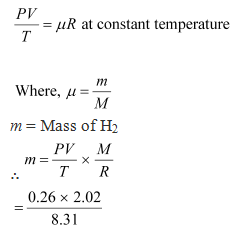= 6.3 × 10–2 g = 6.3 × 10–5 kg
Hence, 6.3 × 10–5 kg of H2 will yield the same value of PV/T.

Qns 13.4: An oxygen cylinder of volume 30 litres has an initial gauge pressure of 15atm and a temperature of 27 °C. After some oxygen is withdrawn from the cylinder, the gauge pressure drops to 11 atm and its temperature drops to 17 °C. Estimate the mass of oxygen taken out of the cylinder (R = 8.31 J mol–1 K–1, molecular mass of O2 = 32 u).
Ans: Volume of oxygen, V1 = 30 litres = 30 × 10–3 m3
Gauge pressure, P1 = 15 atm = 15 × 1.013 × 105 Pa
Temperature, T1 = 27°C = 300 K
Universal gas constant, R = 8.314 J mole–1 K–1
Let the initial number of moles of oxygen gas in the cylinder be n1.
The gas equation is given as: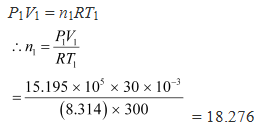But,
Where,
m1 = Initial mass of oxygen
M = Molecular mass of oxygen = 32 g
m1 = n1M = 18.276 × 32 = 584.84 g
After some oxygen is withdrawn from the cylinder, the pressure and temperature reduces.
Volume, V2 = 30 litres = 30 × 10–3 m3
Gauge pressure, P2 = 11 atm = 11 × 1.013 × 105 Pa
Temperature, T2 = 17°C = 290 K
Let n2 be the number of moles of oxygen left in the cylinder.
The gas equation is given as:
P2V2 = n2RT2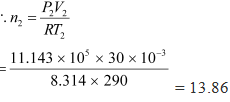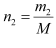But,
Where, m2 is the mass of oxygen remaining in the cylinder
m2 = n2M = 13.86 × 32 = 453.1 g
The mass of oxygen taken out of the cylinder is given by the relation:
Initial mass of oxygen in the cylinder – Final mass of oxygen in the cylinder
= m1m2
= 584.84 g – 453.1 g
= 131.74 g
= 0.131 kg
Therefore, 0.131 kg of oxygen is taken out of the cylinder.

Qns 13.5: An air bubble of volume 1.0 cm3 rises from the bottom of a lake 40 m deep at a temperature of 12 °C. To what volume does it grow when it reaches the surface, which is at a temperature of 35 °C?
Ans: Volume of the air bubble, V1 = 1.0 cm3 = 1.0 × 10–6 m3
Bubble rises to height, d = 40 m
Temperature at a depth of 40 m, T1 = 12°C = 285 K
Temperature at the surface of the lake, T2 = 35°C = 308 K
The pressure on the surface of the lake:
P2 = 1 atm = 1 ×1.013 × 105 Pa
The pressure at the depth of 40 m:
P1 = 1 atm + g
Where,
ρ is the density of water = 103 kg/m3
g is the acceleration due to gravity = 9.8 m/s2
P1 = 1.013 × 105  40 × 103 × 9.8 = 493300 Pa
We have: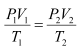Where, V2 is the volume of the air bubble when it reaches the surface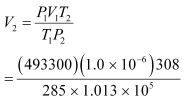= 5.263 × 10–6 m3 or 5.263 cm3

Therefore, when the air bubble reaches the surface, its volume becomes 5.263 cm3.

Qns 13.6: Estimate the total number of air molecules (inclusive of oxygen, nitrogen, water vapour and other constituents) in a room of capacity 25.0 m3 at a temperature of 27 °C and 1 atm pressure.
Ans: Volume of the room, V = 25.0 m3
Temperature of the room, T = 27°C = 300 K
Pressure in the room, P = 1 atm = 1 × 1.013 × 105 Pa
The ideal gas equation relating pressure (P), Volume (V), and absolute temperature (T) can be written as:
PV = kBNT
Where,
KB is Boltzmann constant = 1.38 × 10–23 m2 kg s–2 K–1
N is the number of air molecules in the room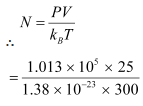= 6.11 × 1026 molecules

Therefore, the total number of air molecules in the given room is 6.11 × 1026.

Qns 13.7: Estimate the average thermal energy of a helium atom at (i) room temperature (27 °C), (ii) the temperature on the surface of the Sun (6000 K), (iii) the temperature of 10 million Kelvin (the typical core temperature in the case of a star).
Ans: (i) At room temperature, T = 27°C = 300 K
Average thermal energy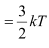Where k is Boltzmann constant = 1.38 × 10–23 m2 kg s–2 K–1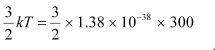= 6.21 × 10–21J

Hence, the average thermal energy of a helium atom at room temperature (27°C) is  6.21 × 10–21 J.
(ii) On the surface of the sun, T = 6000 K
Average thermal energy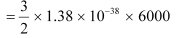= 1.241 × 10–19 J
Hence, the average thermal energy of a helium atom on the surface of the sun is 1.241 × 10–19 J.
(iii) At temperature, T = 107 K
Average thermal energy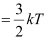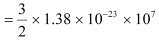= 2.07 × 10–16 J

Hence, the average thermal energy of a helium atom at the core of a star is 2.07 × 10–16 J.

Qns 13.8: Three vessels of equal capacity have gases at the same temperature and pressure. The first vessel contains neon (monatomic), the second contains chlorine (diatomic), and the third contains uranium hexafluoride (polyatomic). Do the vessels contain equal number of respective molecules? Is the root mean square speed of molecules the same in the three cases? If not, in which case is vrms the largest?
Ans: Yes. All contain the same number of the respective molecules.
No. The root mean square speed of neon is the largest.
Since the three vessels have the same capacity, they have the same volume.
Hence, each gas has the same pressure, volume, and temperature.
According to Avogadro’s law, the three vessels will contain an equal number of the respective molecules. This number is equal to Avogadro’s number, N = 6.023 × 1023.
The root mean square speed (vrms) of a gas of mass m, and temperature T, is given by the relation: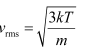Where, k is Boltzmann constant
For the given gases, k and T are constants.
Hence vrms depends only on the mass of the atoms, i.e.,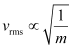Therefore, the root mean square speed of the molecules in the three cases is not the same. Among neon, chlorine, and uranium hexafluoride, the mass of neon is the smallest. Hence, neon has the largest root mean square speed among the given gases.

Qns 13.9: At what temperature is the root mean square speed of an atom in an argon gas cylinder equal to the rms speed of a helium gas atom at – 20 °C? (atomic mass of Ar = 39.9 u, of He = 4.0 u).
Ans: Temperature of the helium atom, THe = –20°C= 253 K
Atomic mass of argon, MAr = 39.9 u
Atomic mass of helium, MHe = 4.0 u
Let, (vrms)Ar be the rms speed of argon.
Let (vrms)He be the rms speed of helium.
The rms speed of argon is given by: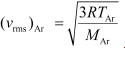......(i)
Where,
R is the universal gas constant
TAr is temperature of argon gas
The rms speed of helium is given by: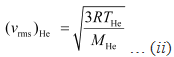It is given that:
(vrms)Ar = (vrms)He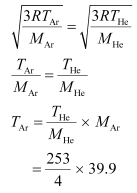= 2523.675 = 2.52 × 103 K
Therefore, the temperature of the argon atom is 2.52 × 103 K.

Qns 13.10: Estimate the mean free path and collision frequency of a nitrogen molecule in a cylinder containing nitrogen at 2.0 atm and temperature 17 °C. Take the radius of a nitrogen molecule to be roughly 1.0 Å. Compare the collision time with the time the molecule moves freely between two successive collisions (Molecular mass of N2 = 28.0 u).
Ans: Mean free path = 1.11 × 10–7 m
Collision frequency = 4.58 × 109 s–1
Successive collision time ≈ 500 × (Collision time)
Pressure inside the cylinder containing nitrogen, P = 2.0 atm = 2.026 × 105 Pa
Temperature inside the cylinder, T = 17°C =290 K
Radius of a nitrogen molecule, r = 1.0 Å = 1 × 1010 m
Diameter, d = 2 × 1 × 1010 = 2 × 1010 m
Molecular mass of nitrogen, M = 28.0 g = 28 × 10–3 kg
The root mean square speed of nitrogen is given by the relation: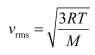Where,
R is the universal gas constant = 8.314 J mole–1 K–1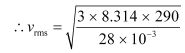= 508.26 m/s
The mean free path (l) is given by the relation: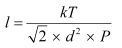Where,
k is the Boltzmann constant = 1.38 × 10–23 kg m2 s–2K–1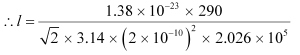= 1.11 × 10–7 m
Collision frequency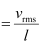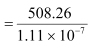= 4.58 × 109 s–1
Collision time is given as: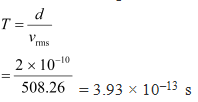= 3.93 × 10–13 s
Time taken between successive collisions: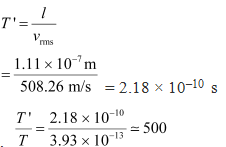= 2.18 × 10–10 s
Hence, the time taken between successive collisions is 500 times the time taken for a collision.

Qns 13.11: A metre long narrow bore held horizontally (and closed at one end) contains a 76 cm long mercury thread, which traps a 15 cm column of air. What happens if the tube is held vertically with the open end at the bottom?
Ans: Length of the narrow bore, L = 1 m = 100 cm
Length of the mercury thread, l = 76 cm
Length of the air column between mercury and the closed end, la = 15 cm
Since the bore is held vertically in air with the open end at the bottom, the mercury length that occupies the air space is: 100 – (76 15) = 9 cm
Hence, the total length of the air column = 15 9 = 24 cm
Let h cm of mercury flow out as a result of atmospheric pressure.
∴ Length of the air column in the bore = 24  h cm
And, length of the mercury column = 76 – h cm
Initial pressure, P1 = 76 cm of mercury
Initial volume, V1 = 15 cm3
Final pressure, P2 = 76 – (76 – h) = h cm of mercury
Final volume, V2 = (24  h) cm3
Temperature remains constant throughout the process.
P1V1 = P2V2
76 × 15 = h (24  h)
h2  24h – 1140 = 0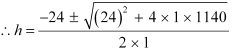= 23.8 cm or –47.8 cm

Height cannot be negative. Hence, 23.8 cm of mercury will flow out from the bore and 52.2 cm of mercury will remain in it. The length of the air column will be 24 23.8 = 47.8 cm.

Qns 13.12: From a certain apparatus, the diffusion rate of hydrogen has an average value of 28.7 cm3 s–1. The diffusion of another gas under the same conditions is measured to have an average rate of 7.2 cm3 s–1. Identify the gas.

[Hint: Use Graham’s law of diffusion: R1/R2 = (M2/M1)1/2, where R1, R2 are diffusion rates of gases 1 and 2, and M1 and M2 their respective molecular masses. The law is a simple consequence of kinetic theory.]
Ans: Rate of diffusion of hydrogen, R1 = 28.7 cm3 s–1
Rate of diffusion of another gas, R2 = 7.2 cm3 s–1
According to Graham’s Law of diffusion, we have: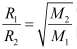Where,
M1 is the molecular mass of hydrogen = 2.020 g
M2 is the molecular mass of the unknown gas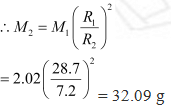32 g is the molecular mass of oxygen. Hence, the unknown gas is oxygen.

Qns 13.13: A gas in equilibrium has uniform density and pressure throughout its volume. This is strictly true only if there are no external influences. A gas column under gravity, for example, does not have uniform density (and pressure). As you might expect, its density decreases with height. The precise dependence is given by the so-called law of atmospheres

n2 = n1 exp [-mg (h2h1)/ kBT]

Where n2, n1 refer to number density at heights h2 and h1 respectively. Use this relation to derive the equation for sedimentation equilibrium of a suspension in a liquid column:

n2 = n1 exp [-mg NA(ρ - P′) (h2h1)/ (ρRT)]

Where ρ is the density of the suspended particle, and ρ’ that of surrounding medium. [NA is Avogadro’s number, and R the universal gas constant.] [Hint: Use Archimedes principle to find the apparent weight of the suspended particle.]

Ans: According to the law of atmospheres, we have:

n2 = n1 exp [-mg (h2h1)/ kBT] … (i)

Where,

n1 is the number density at height h1, and n2 is the number density at height h2

mg is the weight of the particle suspended in the gas column

Density of the medium = ρ'

Density of the suspended particle = ρ

Mass of one suspended particle = m'

Mass of the medium displaced = m

Volume of a suspended particle = V

According to Archimedes’ principle for a particle suspended in a liquid column, the effective weight of the suspended particle is given as:

Weight of the medium displaced – Weight of the suspended particle

= mgm'g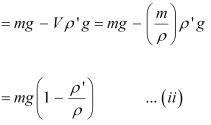Gas constant, R = kBN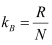.......(iii)

Substituting equation (ii) in place of mg in equation (i) and then using equation (iii), we get:

n2 = n1 exp [-mg (h2h1)/ kBT]

= n1 exp [-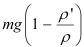(h2h1) ]

= n1 exp [-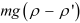(h2h1) ]

Qns 13.14: Given below are densities of some solids and liquids. Give rough estimates of the size of their atoms:

 Substance Atomic Mass (u) Density (103Kg mJ) Carbon (diamond) 12.01 2.22 Gold 197.00 19.32 Nitrogen (liquid) 14.01 1.00 Lithium 6.94 0.53 Fluorine (liquid) 19.00 1.14

[Hint: Assume the atoms to be ‘tightly packed’ in a solid or liquid phase, and use the known value of Avogadro’s number. You should, however, not take the actual numbers you obtain for various atomic sizes too literally. Because of the crudeness of the tight packing approximation, the results only indicate that atomic sizes are in the range of a few Å].

Ans:

 Substance Radius (A) Carbon (diamond) 1.29 Gold 1.59 Nitrogen (liquid) 1.77 Lithium 1.73 Fluorine (liquid) 1.88

Atomic mass of a substance = M

Density of the substance = ρ

Avogadro’s number = N = 6.023 × 1023

Volume of each atom

Volume of N number of molecules  … (i)

Volume of one mole of a substance =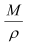… (ii)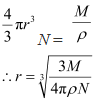For carbon:

M = 12.01 × 10–3 kg,

ρ = 2.22 × 103 kg m–3

= 1.29 Å

Hence, the radius of a carbon atom is 1.29 Å.

For gold:

M = 197.00 × 10–3 kg

ρ = 19.32 × 103 kg m–3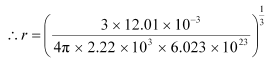= 1.59 Å

Hence, the radius of a gold atom is 1.59 Å.

For liquid nitrogen:

M = 14.01 × 10–3 kg

ρ = 1.00 × 103 kg m–3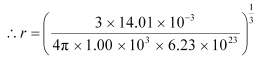= 1.77 Å

Hence, the radius of a liquid nitrogen atom is 1.77 Å.

For lithium:

M = 6.94 × 10–3 kg

ρ = 0.53 × 103 kg m–3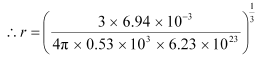= 1.73 Å

Hence, the radius of a lithium atom is 1.73 Å.

For liquid fluorine:

M = 19.00 × 10–3 kg

ρ = 1.14 × 103 kg m–3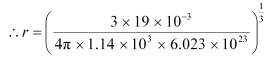= 1.88 Å

Hence, the radius of a liquid fluorine atom is 1.88 Å.

The document Kinetic Theory NCERT Solutions | Physics Class 11 - NEET is a part of the NEET Course Physics Class 11.
All you need of NEET at this link: NEET

## Physics Class 11

124 videos|464 docs|210 tests

## Physics Class 11

124 videos|464 docs|210 tests

### How to Prepare for NEET

Read our guide to prepare for NEET which is created by Toppers & the best Teachers

Track your progress, build streaks, highlight & save important lessons and more!(Scan QR code)

,

,

,

,

,

,

,

,

,

,

,

,

,

,

,

,

,

,

,

,

,

;